# The MatPlotLib Python Plotting Library¶

## Perquisites¶

Before looking at any Python library it is recommended to familarise yourself with the Python programming language and object orientated programming concepts. Details about these in addition to Python installation instructions are available in my perquisite guide below.

It is also helpful to understand the numeric python library and the Python and data analysis libraries as we will use these to create data to plot from.

## Importing the matplotlib library¶

To get started with matplotlib we will need the library numpy, pandas and the pyplot module from the matplotlib library.

In :
import numpy as np
import pandas as pd
import matplotlib.pyplot as plt


## Creating Data¶

Two lists x (time s) and y (velocity m/s) will be used to demonstrate basic 2D plots. They are of equal length.

In :
x=[0,10,15,20,22.5,30]
y=[0,227.04,362.78,517.35,602.97,901.67]

In :
x

Out:
[0, 10, 15, 20, 22.5, 30]
In :
y

Out:
[0, 227.04, 362.78, 517.35, 602.97, 901.67]

These can be converted into numpy arrays.

In :
xa=np.array(x)

In :
ya=np.array(y)

In :
xa

Out:
array([ 0. , 10. , 15. , 20. , 22.5, 30. ])
In :
ya

Out:
array([  0.  , 227.04, 362.78, 517.35, 602.97, 901.67])

Also x can be converted into a pandas series.

In :
se=pd.Series(x)


Then converted to a dataframe.

In :
df=pd.DataFrame(se,columns=['x'])


y can be added to this dataframe.

In :
df['y']=y

In :
df

Out:
x y
0 0.0 0.00
1 10.0 227.04
2 15.0 362.78
3 20.0 517.35
4 22.5 602.97
5 30.0 901.67

## Specifying the Plot Backend¶

IPython has a number of line magic functions that follow command line syntax and begin with a %. The matplotlib magic line fucntion can be used to specify how plots behave. If we type it in followed by a shift ⇧ and tab ↹ we will get the docstring.There are two main options:

• inline which creates an inline (static image) which will display as an output in a cell.
• auto which creates an interactive plot in a seperate window. There is a third option but requires installation of a third party extension:
• widget which creates an interactive image which will display as an output in a cell.

### inline¶

In :
%matplotlib inline
plt.plot(x,y)

Out:
[<matplotlib.lines.Line2D at 0x2bede20ebb0>]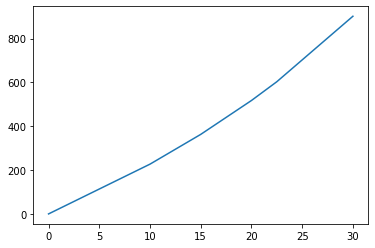Alternatively to plot from the dataframe one can use.

In :
%matplotlib inline
plt.plot(df.x,df.y)

Out:
[<matplotlib.lines.Line2D at 0x2bede97ebe0>]### auto¶

In :
%matplotlib auto
plt.plot(x,y)

Using matplotlib backend: Qt5Agg

Out:
[<matplotlib.lines.Line2D at 0x2bee06a8e80>]

The output of the cell will inform you that there is matplolib plot using the Qt5Agg backend.The user can switch seamlessly between inline and auto within a single workbook.

### widget¶

Switching between auto and widget requires a restart of the Kernel however once widget is selected on a fresh kernel the user can switch between widget and inline.

%matplotlib widget

plt.plot(x,y)## MATLAB syntax¶

The pyplot module of the matplotlib library has two types of syntax. The first syntax is designed to have MATLAB like syntax which is a popular commercial program within mathematics and scientific fields. The pyplot matlab syntax can be used to quickly generate basic 2D plots however this syntax lacks behind MATLAB when it comes to 3D plots and animations. The pyplot module also uses object orientated programming syntax which is required to do advanced plots.

### Figures¶

Every function from the MATLAB syntax is directly selected from the pyplot module. Type in plt followed by a dot . and tab ↹ to view the commands available.To view all of these the function dir can be used with plt as the positional input argument.

In :
dir(plt)

Out:
['Annotation',
'Arrow',
'Artist',
'AutoLocator',
'Axes',
'Button',
'Circle',
'Figure',
'FigureCanvasBase',
'FixedFormatter',
'FixedLocator',
'FormatStrFormatter',
'Formatter',
'FuncFormatter',
'GridSpec',
'IndexLocator',
'Line2D',
'LinearLocator',
'Locator',
'LogFormatter',
'LogFormatterExponent',
'LogFormatterMathtext',
'LogLocator',
'MaxNLocator',
'MouseButton',
'MultipleLocator',
'Normalize',
'NullFormatter',
'NullLocator',
'Number',
'PolarAxes',
'Polygon',
'Rectangle',
'ScalarFormatter',
'Slider',
'Subplot',
'SubplotTool',
'Text',
'TickHelper',
'Widget',
'_INSTALL_FIG_OBSERVER',
'_IP_REGISTERED',
'__builtins__',
'__cached__',
'__doc__',
'__file__',
'__name__',
'__package__',
'__spec__',
'_auto_draw_if_interactive',
'_backend_mod',
'_code_objs',
'_copy_docstring_and_deprecators',
'_get_required_interactive_framework',
'_interactive_bk',
'_log',
'_pylab_helpers',
'_setup_pyplot_info_docstrings',
'_xkcd',
'acorr',
'angle_spectrum',
'annotate',
'arrow',
'autoscale',
'autumn',
'axes',
'axhline',
'axhspan',
'axis',
'axline',
'axvline',
'axvspan',
'bar',
'barbs',
'barh',
'bone',
'box',
'boxplot',
'broken_barh',
'cbook',
'cla',
'clabel',
'clf',
'clim',
'close',
'cm',
'cohere',
'colorbar',
'colormaps',
'connect',
'contour',
'contourf',
'cool',
'copper',
'csd',
'cycler',
'delaxes',
'disconnect',
'docstring',
'draw',
'draw_all',
'draw_if_interactive',
'errorbar',
'eventplot',
'figaspect',
'figimage',
'figlegend',
'fignum_exists',
'figtext',
'figure',
'fill',
'fill_between',
'fill_betweenx',
'findobj',
'flag',
'functools',
'gca',
'gcf',
'gci',
'get',
'get_backend',
'get_cmap',
'get_current_fig_manager',
'get_figlabels',
'get_fignums',
'get_plot_commands',
'get_scale_names',
'getp',
'ginput',
'gray',
'grid',
'hexbin',
'hist',
'hist2d',
'hlines',
'hot',
'hsv',
'importlib',
'imsave',
'imshow',
'inferno',
'inspect',
'install_repl_displayhook',
'interactive',
'ioff',
'ion',
'isinteractive',
'jet',
'legend',
'locator_params',
'logging',
'loglog',
'magma',
'magnitude_spectrum',
'margins',
'matplotlib',
'matshow',
'minorticks_off',
'minorticks_on',
'mlab',
'new_figure_manager',
'nipy_spectral',
'np',
'pause',
'pcolor',
'pcolormesh',
'phase_spectrum',
'pie',
'pink',
'plasma',
'plot',
'plot_date',
'plotting',
'polar',
'prism',
'psd',
'quiver',
'quiverkey',
'rc',
'rcParams',
'rcParamsDefault',
'rcParamsOrig',
'rc_context',
'rcdefaults',
'rcsetup',
're',
'register_cmap',
'rgrids',
'savefig',
'sca',
'scatter',
'sci',
'semilogx',
'semilogy',
'set_cmap',
'set_loglevel',
'setp',
'show',
'specgram',
'spring',
'spy',
'stackplot',
'stem',
'step',
'streamplot',
'style',
'subplot',
'subplot2grid',
'subplot_mosaic',
'subplot_tool',
'subplots',
'summer',
'suptitle',
'switch_backend',
'sys',
'table',
'text',
'thetagrids',
'tick_params',
'ticklabel_format',
'tight_layout',
'time',
'title',
'tricontour',
'tricontourf',
'tripcolor',
'triplot',
'twinx',
'twiny',
'uninstall_repl_displayhook',
'violinplot',
'viridis',
'vlines',
'waitforbuttonpress',
'winter',
'xcorr',
'xkcd',
'xlabel',
'xlim',
'xscale',
'xticks',
'ylabel',
'ylim',
'yscale',
'yticks']

The functions of interest at the moment are the following.

figure axes plot
figure axis plot
show gca scatter
imshow subplot axhline
savefig xlabel axvline
close ylabel
clf title grid
xlim legend
ylim
xscale
yscale
xticks
yticks

We can think of the list to the left as being related to the figure, the list to the middle being related to the axes and the list to the right being different plot types. The workflow usually involves setting a figure, then adding an axes to the figure and finally adding a figure to those axes.

#### figure¶

The window title in the automatic backend displays the figure number. We can assign the figure number by using the figure function. Note the keyword input arguments num (also acts as a positional input argument) has a default value of None. When set to None, the num will be assigned the next unused figure. The figsize can be a tuple containing the width and height in inches and the dots per inch dpi can be an integer. Both these keyword input have the default None which corresponds to 6 inches by 4 inches and 72 dpi respectively. We will leave all the rest at default.Note when a new cell is ran, any auto figures open from previous cells will close. If we type in the following the lowest unused Figure (starting at 1) will be created as a new window showing a new blank canvas. In this case because there are no figures open it will displaya s Figure 1.

In :
%matplotlib auto
plt.figure(num=1)

Using matplotlib backend: Qt5Agg

Out:
<Figure size 432x288 with 0 Axes>Alternatively we can go straight to figure 100 and assign a wider figure size using the figsize keyword argument.

In :
%matplotlib auto
plt.figure(num=100,figsize=(12,3))

Using matplotlib backend: Qt5Agg

Out:
<Figure size 864x216 with 0 Axes>We can create multiple figures be specifying them in a single cell. Note the third figure will be figure 101 (the next figure after the highest number 100).

In :
%matplotlib auto
plt.figure(num=1)
plt.figure(100,figsize=(12,3))
plt.figure()

Using matplotlib backend: Qt5Agg

Out:
<Figure size 432x288 with 0 Axes>Any plot will be plotted on the currently selected figure.

In :
%matplotlib auto
plt.figure(num=1)
plt.plot(x,ya,color='r')
plt.plot(x,2*ya,color='k')
plt.figure(100,figsize=(12,3))
plt.plot(x,y,color='b')
plt.figure()
plt.plot(x,y,color='g')

Using matplotlib backend: Qt5Agg

Out:
[<matplotlib.lines.Line2D at 0x2bee074c4c0>]#### savefig¶

We can use the function savefig to save the figure to a png file. By default it will be saved in the same folder as the jupyterlab notebook.

In :
%matplotlib auto
plt.figure(num=1)
plt.plot(x,y,color='r')
plt.savefig('fig1_saved')

Using matplotlib backend: Qt5Agg#### close¶

We can use the function close the figure. This will open and close the figure immediately after its created. For example if we only want to save the figure and not view it.

In :
%matplotlib auto
plt.figure(num=2)
plt.plot(x,y,color='k')
plt.savefig('fig2_saved')
plt.close()

Using matplotlib backend: Qt5AggNote if the figure is colsed before it is saved, the previous plot on it will be lost.

In :
%matplotlib auto
plt.figure(num=3)
plt.plot(x,y,color='g')
plt.close()
plt.savefig('fig3_saved')

Using matplotlib backend: Qt5Agg#### show¶

Plots display as outputs from cells by default.

Notice however the output of the cell shows at the top "Text(0.5, 0, 'time (s)')" which corresponds to the text in the last function xlabel.

In :
%matplotlib inline
plt.figure()
plt.plot(x,y,color='g')
plt.xlabel('time (s)')

Out:
Text(0.5, 0, 'time (s)')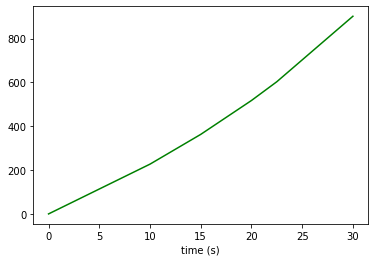To prevent this we can end the cell using the function show. To get details about the keyword input arguments press shift ⇧ and tab ↹. We will leave these at their defaults.In :
%matplotlib inline
plt.figure()
plt.plot(x,y,color='g')
plt.xlabel('time (s)')
plt.show()#### clf¶

The clf function can be used to clear the figure.Notice that the green line and the xlabel are cleared from the plto and only the blue line displays.

In :
%matplotlib inline
plt.figure()
plt.plot(x,y,color='g')
plt.xlabel('time (s)')
plt.clf()
plt.plot(df.x,2*df.y,color='b')
plt.show()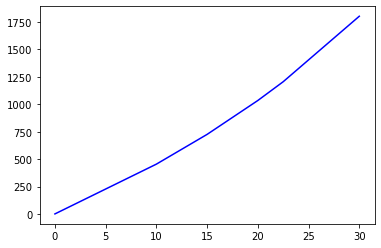### Axes¶

#### subplot¶

Notice in the case above the last three commands are in matlab style, the figure is selected and the operations from any of the other functions called directly from the module plt will act on the existing figure. When we used the function plot, axes were created for us by default taking up the full canvas of the graph. It is possible to explicitly create axes using the function subplot. Calling the function from the pyplot module and pressing shift ⇧ and tab ↹ displays the positional input arguments. There are three nrows, ncols and index which are all 1 by default.In :
%matplotlib auto
plt.figure(num=4)
plt.subplot(1,1,1)
plt.plot(x,y,color='g')

Using matplotlib backend: Qt5Agg

Out:
[<matplotlib.lines.Line2D at 0x2bee086c5e0>]If we instead specify 2 rows and 1 column we will have 2*1=2 indexes to plot on (index 1 and 2).

In :
%matplotlib auto
plt.figure(num=5)
plt.subplot(2,1,1)
plt.plot(xa,ya,color='k')
plt.plot(xa,2*ya,color='b')
plt.subplot(2,1,2)
plt.plot(x,y,color='r')

Using matplotlib backend: Qt5Agg

Out:
[<matplotlib.lines.Line2D at 0x2bee08dbdc0>]Note when using subplot the index goes along rows and then down to the next column (similar to a document).

In :
%matplotlib auto
plt.figure(num=6)
plt.subplot(2,2,1)
plt.plot(xa,ya,color='k')
plt.plot(xa,2*ya,color='b')
plt.subplot(2,2,2)
plt.plot(xa,ya,color='m')
plt.subplot(2,2,3)
plt.plot(xa,ya,color='r')

Using matplotlib backend: Qt5Agg

Out:
[<matplotlib.lines.Line2D at 0x2bee098c6d0>]#### xlabel, ylabel and title¶

So far our figure axes have been unlabelled. We can label them by using the functions xlabel, ylabel and title respectively. Typing in one of the functions and pressing shift ⇧ and tab ↹ will display the input arguments. We see we have a postional input argument which is the label in the form of a str. We also have a number of keyword input arguments related to the font and location. These are both set to a default of None meaning default fonts and positions are used.In :
%matplotlib auto
plt.figure(num=7)
plt.plot(xa,ya,color='r')
plt.xlabel('time (s)')
plt.ylabel('velocity (m/s)')
plt.title('rocket projectile')

Using matplotlib backend: Qt5Agg

Out:
Text(0.5, 1.0, 'rocket projectile')Note that the output of the cell displays at the top details about the last text entry 'rocket projectile' which is the title. The title is centred in x (shown as a normalized float of 0.5; left is 0 and right is 1) and to the top of the chart (shown as a normalized float of 1; the bottom is 0 and the top is 1).

A font dict can be prepared which includes the keys family, color, weight and size.

In :
font = {'family':'Arial',
'color':'m',
'weight':'bold',
'size':18,
}


The keyword input arguments fontdict can be assigned to the font dict and the keyword input loc can be assigned to the right. Let's use xlabel as the last line so its details display.

In :
%matplotlib auto
plt.figure(num=7)
plt.plot(xa,ya,color='r')
plt.ylabel('velocity (m/s)')
plt.title('rocket projectile')
plt.xlabel('time (s)')

Using matplotlib backend: Qt5Agg

Out:
Text(0.5, 0, 'time (s)')We see that the Text(0.5, 0, 'time (s)') displays denoting that xlabel is halfway along in x and at the bottom in y.

In :
%matplotlib auto
plt.figure(num=8)
plt.plot(xa,ya,color='r')
plt.ylabel('velocity (m/s)')
plt.title('rocket projectile')
plt.xlabel('time (s)',fontdict=font,loc='right')

Using matplotlib backend: Qt5Agg

Out:
Text(1, 0, 'time (s)')We see that the Text(1, 0, 'time (s)') displays denoting that xlabel is to the right in x and at the bottom in y.

#### xlim and ylim¶

We can change the limits of the axes usign the functions xlim and ylim. Once again we can press shift ⇧ and tab ↹ to get details about the input arguments. Both functions are quite similar as expected. They can either have a tuple with the left and right limits or bottom and top limits respectively. Or use the keyword input arguments left and right or up and down respectively.In :
%matplotlib auto
plt.figure(num=9)
plt.plot(xa,ya,color='r')
plt.xlabel('time (s)')
plt.xlim(left=0,right=20)
plt.ylabel('velocity (m/s)')
plt.ylim(top=500)
plt.title('rocket projectile')

Using matplotlib backend: Qt5Agg

Out:
Text(0.5, 1.0, 'rocket projectile')
In :
%matplotlib auto
plt.figure(num=9)
plt.plot(xa,ya,color='r')
plt.xlabel('time (s)')
plt.xlim(left=0,right=20)
plt.ylabel('velocity (m/s)')
plt.ylim(top=500)
plt.title('rocket projectile')

Using matplotlib backend: Qt5Agg

Out:
Text(0.5, 1.0, 'rocket projectile')#### xscale and yscale¶

The functions xscale and yscale can be used to change the scale of the axes. Once again we can type in the functions name and press shift ⇧ and tab ↹ to get details about the input arguments. We see that the defautl value is the str 'linear' and this can be changed to 'log' (used to emphasize small numbers and display exponential data. The log of an exponential is a straight line), 'symlog' (a log scale which handles negative values) and 'logit' (a statistical probability scale).Let's change the y-scale to log for example.

In :
%matplotlib auto
plt.figure(num=10)
plt.plot(xa,ya,color='r')
plt.xlabel('time (s)')
plt.ylabel('log velocity (m/s)')
plt.yscale('log')
plt.title('rocket projectile')

Using matplotlib backend: Qt5Agg

Out:
Text(0.5, 1.0, 'rocket projectile')#### xticks and yticks¶

The functions xticks and yticks can be used to change the location of the ticks. Once again we can type in the functions name and then press shift ⇧ and tab ↹ to get details about the input arguments. We see we have the keyword input arguments ticks which is a list of numeric datapoints we wish to see the ticks. We also have the list labels which can be a list of str of equal length which will display instead of the numeric values.Let's switch to inline plotting.

In :
%matplotlib inline
plt.plot(xa,ya,color='r')
plt.xlabel('time (s)')
plt.ylabel('velocity (m/s)')
plt.title('rocket projectile')

Out:
Text(0.5, 1.0, 'rocket projectile')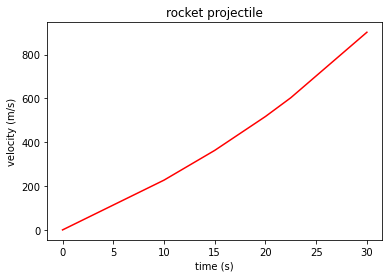We see that the xticks are spaced using every 5 s. If we want them to instead display every 10 s we can use the following list tickx. We can then use the keyword input argument ticks and assign it to the list.

In :
tickx=[0,10,20,30]

%matplotlib inline
plt.plot(xa,ya,color='r')
plt.xlabel('time (s)')
plt.xticks(ticks=tickx)
plt.ylabel('velocity (m/s)')
plt.title('rocket projectile')

Out:
Text(0.5, 1.0, 'rocket projectile')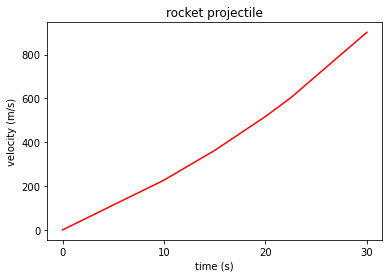If we instead wanted labels instead of the numbers we could specify a list of str of equal size labelx. We can then use the keyword input argument labels and assign it to the list.

In :
tickx=[0,10,20,30]
labelx=['zero','ten','twenty','thirty']

%matplotlib inline
plt.plot(xa,ya,color='r')
plt.xlabel('time (s)')
plt.xticks(ticks=tickx,labels=labelx)
plt.ylabel('velocity (m/s)')
plt.title('rocket projectile')

Out:
Text(0.5, 1.0, 'rocket projectile')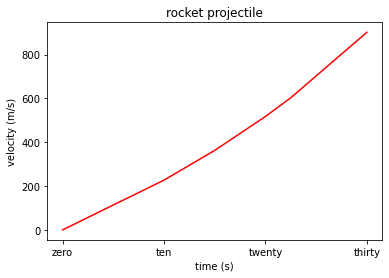Note the top of the cell output shows details about the last statement in this case that it is text that is at an x position of 0.5 (a float normalized to 1; left of the plot is 0, right of the plot is 1) and a y position of 1 (another float normalized to 1; bottom of the plot is 0 and top of the plot is 1).

#### text and annotate¶

We can use the function text to place custom text. To get details baout the input argumetns we can press shift ⇧ and tab ↹. We have the positional input arguments x an y which this time refer to the starting position of the str on the x and y axes respectively. The positional input argument s is the str we want to add. We can also use a fontdict as seen with xlabel, ylabel and title.In :
%matplotlib inline
plt.plot(xa,ya,color='r')
plt.xlabel('time (s)')
tickx=[0,10,20,30]
plt.xticks(ticks=tickx)
plt.ylabel('velocity (m/s)')
plt.title('rocket projectile')
font = {'family':'Arial',
'color':'m',
'weight':'bold',
'size':18,
}
plt.text(10,600,'Custom Text',fontdict=font)

Out:
Text(10, 600, 'Custom Text')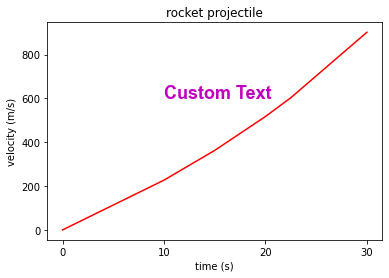Another related function is annotate which allows us to place text at a certain position and draw a line from the text to a datapoint to highlight it.

• The keyword input argument xytext is a (x_start,y_start) tuple specifying where the keyword input argument text should begin.
• The keyword input argument xy is a (x_end,y_end) tuple specifying the datapoint to be annotated. The dictionary arrowprops must be specified as a dictionary to draw an arrow.

The arrow properties such as the arrowstyle and connectionstyle must be specified as key value pairs in the dictionary.The docstring is quite long so we can view it in the output of a cell using.

In :
? plt.annotate

Signature:  plt.annotate(text, xy, *args, **kwargs)
Docstring:
Annotate the point *xy* with text *text*.

In the simplest form, the text is placed at *xy*.

Optionally, the text can be displayed in another position *xytext*.
An arrow pointing from the text to the annotated point *xy* can then

Parameters
----------
text : str
The text of the annotation.  *s* is a deprecated synonym for this
parameter.

xy : (float, float)
The point *(x, y)* to annotate. The coordinate system is determined
by *xycoords*.

xytext : (float, float), default: *xy*
The position *(x, y)* to place the text at. The coordinate system
is determined by *textcoords*.

xycoords : str or .Artist or .Transform or callable or (float, float), default: 'data'

The coordinate system that *xy* is given in. The following types
of values are supported:

- One of the following strings:

=================   =============================================
Value               Description
=================   =============================================
'figure points'     Points from the lower left of the figure
'figure pixels'     Pixels from the lower left of the figure
'figure fraction'   Fraction of figure from lower left
'axes points'       Points from lower left corner of axes
'axes pixels'       Pixels from lower left corner of axes
'axes fraction'     Fraction of axes from lower left
'data'              Use the coordinate system of the object being
annotated (default)
'polar'             *(theta, r)* if not native 'data' coordinates
=================   =============================================

- An .Artist: *xy* is interpreted as a fraction of the artist's
~matplotlib.transforms.Bbox. E.g. *(0, 0)* would be the lower
left corner of the bounding box and *(0.5, 1)* would be the
center top of the bounding box.

- A .Transform to transform *xy* to screen coordinates.

- A function with one of the following signatures::

def transform(renderer) -> Bbox
def transform(renderer) -> Transform

where *renderer* is a .RendererBase subclass.

The result of the function is interpreted like the .Artist and
.Transform cases above.

- A tuple *(xcoords, ycoords)* specifying separate coordinate
systems for *x* and *y*. *xcoords* and *ycoords* must each be
of one of the above described types.

See :ref:plotting-guide-annotation for more details.

textcoords : str or .Artist or .Transform or callable or (float, float), default: value of *xycoords*
The coordinate system that *xytext* is given in.

All *xycoords* values are valid as well as the following
strings:

=================   =========================================
Value               Description
=================   =========================================
'offset points'     Offset (in points) from the *xy* value
'offset pixels'     Offset (in pixels) from the *xy* value
=================   =========================================

arrowprops : dict, optional
The properties used to draw a .FancyArrowPatch arrow between the
positions *xy* and *xytext*.

If *arrowprops* does not contain the key 'arrowstyle' the
allowed keys are:

==========   ======================================================
Key          Description
==========   ======================================================
width        The width of the arrow in points
headwidth    The width of the base of the arrow head in points
shrink       Fraction of total length to shrink from both ends
?            Any key to :class:matplotlib.patches.FancyArrowPatch
==========   ======================================================

If *arrowprops* contains the key 'arrowstyle' the
above keys are forbidden.  The allowed values of
'arrowstyle' are:

============   =============================================
Name           Attrs
============   =============================================
'-'        None
'->'       head_length=0.4,head_width=0.2
'-['       widthB=1.0,lengthB=0.2,angleB=None
'|-|'      widthA=1.0,widthB=1.0
'-|>'      head_length=0.4,head_width=0.2
'<-'       head_length=0.4,head_width=0.2
'<->'      head_length=0.4,head_width=0.2
'<|-'      head_length=0.4,head_width=0.2
'<|-|>'    head_length=0.4,head_width=0.2
'fancy'    head_length=0.4,head_width=0.4,tail_width=0.4
'simple'   head_length=0.5,head_width=0.5,tail_width=0.2
'wedge'    tail_width=0.3,shrink_factor=0.5
============   =============================================

Valid keys for ~matplotlib.patches.FancyArrowPatch are:

===============  ==================================================
Key              Description
===============  ==================================================
arrowstyle       the arrow style
connectionstyle  the connection style
relpos           default is (0.5, 0.5)
patchA           default is bounding box of the text
patchB           default is None
shrinkA          default is 2 points
shrinkB          default is 2 points
mutation_scale   default is text size (in points)
mutation_aspect  default is 1.
?                any key for :class:matplotlib.patches.PathPatch
===============  ==================================================

Defaults to None, i.e. no arrow is drawn.

annotation_clip : bool or None, default: None
Whether to draw the annotation when the annotation point *xy* is
outside the axes area.

- If *True*, the annotation will only be drawn when *xy* is
within the axes.
- If *False*, the annotation will always be drawn.
- If *None*, the annotation will only be drawn when *xy* is
within the axes and *xycoords* is 'data'.

**kwargs
Additional kwargs are passed to ~matplotlib.text.Text.

Returns
-------
.Annotation

--------
:ref:plotting-guide-annotation
File:      c:\users\phili\anaconda3\lib\site-packages\matplotlib\pyplot.py
Type:      function

In :
%matplotlib inline
plt.plot(xa,ya,color='r')
plt.xlabel('time (s)')
tickx=[0,10,20,30]
plt.xticks(ticks=tickx)
plt.ylabel('velocity (m/s)')
plt.title('rocket projectile')

arrowproperties={'arrowstyle':'->',
'connectionstyle':'arc'}
plt.annotate(text='Custom Text',xytext=(20,200),
arrowprops=arrowproperties,xy=(10,100))

Out:
Text(20, 200, 'Custom Text')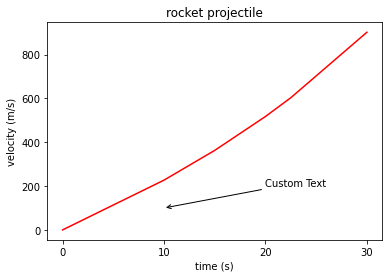Here we see that the line begins from the Custom Text, which begins at (20,200) and points towards the specified point at (10,100).

We can update this to point at a certain data point. Let's change xy to (20,517.35).

In :
%matplotlib inline
plt.plot(xa,ya,color='r')
plt.xlabel('time (s)')
tickx=[0,10,20,30]
plt.xticks(ticks=tickx)
plt.ylabel('velocity (m/s)')
plt.title('rocket projectile')

arrowproperties={'arrowstyle':'->',
'connectionstyle':'arc3'}
plt.annotate(text='Data Point',xytext=(20,200),
arrowprops=arrowproperties,xy=(20,517.35))

Out:
Text(20, 200, 'Data Point')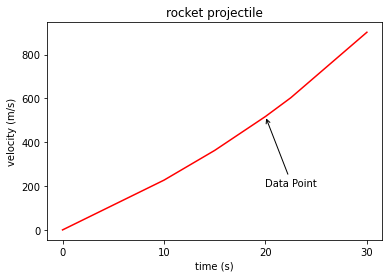We used a straight line above. We can specify a rounding radius of the edge using the keyword input argument rad (rad=0 is a straight line) to instead get an arc.

In :
%matplotlib inline
plt.plot(xa,ya,color='r')
plt.xlabel('time (s)')
tickx=[0,10,20,30]
plt.xticks(ticks=tickx)
plt.ylabel('velocity (m/s)')
plt.title('rocket projectile')

arrowproperties={'arrowstyle':'->',
plt.annotate(text='Data Point',xytext=(20,200),
arrowprops=arrowproperties,xy=(20,517.35))
arrowproperties={'arrowstyle':'->',
plt.annotate(text='Data Point',xytext=(20,200),
arrowprops=arrowproperties,xy=(20,517.35))
arrowproperties={'arrowstyle':'->',
plt.annotate(text='Data Point',xytext=(20,200),
arrowprops=arrowproperties,xy=(20,517.35))

Out:
Text(20, 200, 'Data Point')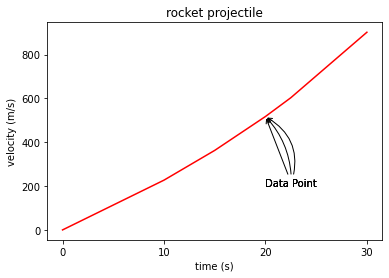We can change the connection style from arc to angle. When we use angle, we need to specify angleA and angleB.

• angleA occurs from the text (if we add a horizontal line using axhline) we can see it is 35 degrees above the horizontal line (+35).
• angleB occurs from the datapoint (if we add a horizontal line using axhline) we can see it is 35 degrees below the horizontal line (-35).
In :
%matplotlib inline
plt.plot(xa,ya,color='r')
plt.xlabel('time (s)')
tickx=[0,10,20,30]
plt.xticks(ticks=tickx)
plt.ylabel('velocity (m/s)')
plt.title('rocket projectile')

arrowproperties={'arrowstyle':'->',
'connectionstyle':'angle3,angleA=35,angleB=-35'}
plt.annotate(text='Data Point',xytext=(20,200),
arrowprops=arrowproperties,xy=(20,517.35))
plt.axhline(y=200,color='#000000',linestyle=':',linewidth=0.5)
plt.axhline(y=517.35,color='#000000',linestyle=':',linewidth=0.5)

Out:
<matplotlib.lines.Line2D at 0x2bee0cf3c40>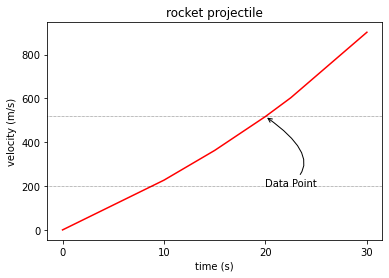### Plots¶

#### plot¶

plot is a function sued to create a 2D line plot.

We have used the function plot but haven't yet explored its input arguments. Once again we can type in the function name and then press shift ⇧ and tab ↹ to get details about the input arguments.We see that the scroll bar for the docstring is pretty long. We can get the full output in a cell using.

In :
? plt.plot

Signature:  plt.plot(*args, scalex=True, scaley=True, data=None, **kwargs)
Docstring:
Plot y versus x as lines and/or markers.

Call signatures::

plot([x], y, [fmt], *, data=None, **kwargs)
plot([x], y, [fmt], [x2], y2, [fmt2], ..., **kwargs)

The coordinates of the points or line nodes are given by *x*, *y*.

The optional parameter *fmt* is a convenient way for defining basic
formatting like color, marker and linestyle. It's a shortcut string
notation described in the *Notes* section below.

>>> plot(x, y)        # plot x and y using default line style and color
>>> plot(x, y, 'bo')  # plot x and y using blue circle markers
>>> plot(y)           # plot y using x as index array 0..N-1
>>> plot(y, 'r+')     # ditto, but with red plusses

You can use .Line2D properties as keyword arguments for more
control on the appearance. Line properties and *fmt* can be mixed.
The following two calls yield identical results:

>>> plot(x, y, 'go--', linewidth=2, markersize=12)
>>> plot(x, y, color='green', marker='o', linestyle='dashed',
...      linewidth=2, markersize=12)

When conflicting with *fmt*, keyword arguments take precedence.

**Plotting labelled data**

There's a convenient way for plotting objects with labelled data (i.e.
data that can be accessed by index obj['y']). Instead of giving
the data in *x* and *y*, you can provide the object in the *data*
parameter and just give the labels for *x* and *y*::

>>> plot('xlabel', 'ylabel', data=obj)

All indexable objects are supported. This could e.g. be a dict, a
pandas.DataFrame or a structured numpy array.

**Plotting multiple sets of data**

There are various ways to plot multiple sets of data.

- The most straight forward way is just to call plot multiple times.
Example:

>>> plot(x1, y1, 'bo')
>>> plot(x2, y2, 'go')

- Alternatively, if your data is already a 2d array, you can pass it
directly to *x*, *y*. A separate data set will be drawn for every
column.

Example: an array a where the first column represents the *x*
values and the other columns are the *y* columns::

>>> plot(a, a[1:])

- The third way is to specify multiple sets of *[x]*, *y*, *[fmt]*
groups::

>>> plot(x1, y1, 'g^', x2, y2, 'g-')

In this case, any additional keyword argument applies to all
datasets. Also this syntax cannot be combined with the *data*
parameter.

By default, each line is assigned a different style specified by a
'style cycle'. The *fmt* and line property parameters are only
necessary if you want explicit deviations from these defaults.
Alternatively, you can also change the style cycle using
:rc:axes.prop_cycle.

Parameters
----------
x, y : array-like or scalar
The horizontal / vertical coordinates of the data points.
*x* values are optional and default to range(len(y)).

Commonly, these parameters are 1D arrays.

They can also be scalars, or two-dimensional (in that case, the
columns represent separate data sets).

These arguments cannot be passed as keywords.

fmt : str, optional
A format string, e.g. 'ro' for red circles. See the *Notes*
section for a full description of the format strings.

Format strings are just an abbreviation for quickly setting
basic line properties. All of these and more can also be
controlled by keyword arguments.

This argument cannot be passed as keyword.

data : indexable object, optional
An object with labelled data. If given, provide the label names to
plot in *x* and *y*.

.. note::
Technically there's a slight ambiguity in calls where the
second label is a valid *fmt*. plot('n', 'o', data=obj)
could be plt(x, y) or plt(y, fmt). In such cases,
the former interpretation is chosen, but a warning is issued.
You may suppress the warning by adding an empty format string
plot('n', 'o', '', data=obj).

Returns
-------
list of .Line2D
A list of lines representing the plotted data.

Other Parameters
----------------
scalex, scaley : bool, default: True
These parameters determine if the view limits are adapted to the
data limits. The values are passed on to autoscale_view.

**kwargs : .Line2D properties, optional
*kwargs* are used to specify properties like a line label (for
auto legends), linewidth, antialiasing, marker face color.
Example::

>>> plot([1, 2, 3], [1, 2, 3], 'go-', label='line 1', linewidth=2)
>>> plot([1, 2, 3], [1, 4, 9], 'rs', label='line 2')

If you make multiple lines with one plot call, the kwargs
apply to all those lines.

Here is a list of available .Line2D properties:

Properties:
agg_filter: a filter function, which takes a (m, n, 3) float array and a dpi value, and returns a (m, n, 3) array
alpha: float or None
animated: bool
antialiased or aa: bool
clip_box: .Bbox
clip_on: bool
clip_path: Patch or (Path, Transform) or None
color or c: color
contains: unknown
dash_capstyle: {'butt', 'round', 'projecting'}
dash_joinstyle: {'miter', 'round', 'bevel'}
dashes: sequence of floats (on/off ink in points) or (None, None)
data: (2, N) array or two 1D arrays
drawstyle or ds: {'default', 'steps', 'steps-pre', 'steps-mid', 'steps-post'}, default: 'default'
figure: .Figure
fillstyle: {'full', 'left', 'right', 'bottom', 'top', 'none'}
gid: str
in_layout: bool
label: object
linestyle or ls: {'-', '--', '-.', ':', '', (offset, on-off-seq), ...}
linewidth or lw: float
marker: marker style string, ~.path.Path or ~.markers.MarkerStyle
markeredgecolor or mec: color
markeredgewidth or mew: float
markerfacecolor or mfc: color
markerfacecoloralt or mfcalt: color
markersize or ms: float
markevery: None or int or (int, int) or slice or List[int] or float or (float, float) or List[bool]
path_effects: .AbstractPathEffect
picker: unknown
rasterized: bool or None
sketch_params: (scale: float, length: float, randomness: float)
snap: bool or None
solid_capstyle: {'butt', 'round', 'projecting'}
solid_joinstyle: {'miter', 'round', 'bevel'}
transform: matplotlib.transforms.Transform
url: str
visible: bool
xdata: 1D array
ydata: 1D array
zorder: float

--------
scatter : XY scatter plot with markers of varying size and/or color (
sometimes also called bubble chart).

Notes
-----
**Format Strings**

A format string consists of a part for color, marker and line::

fmt = '[marker][line][color]'

Each of them is optional. If not provided, the value from the style
cycle is used. Exception: If line is given, but no marker,
the data will be a line without markers.

Other combinations such as [color][marker][line] are also
supported, but note that their parsing may be ambiguous.

**Markers**

=============    ===============================
character        description
=============    ===============================
'.'          point marker
','          pixel marker
'o'          circle marker
'v'          triangle_down marker
'^'          triangle_up marker
'<'          triangle_left marker
'>'          triangle_right marker
'1'          tri_down marker
'2'          tri_up marker
'3'          tri_left marker
'4'          tri_right marker
's'          square marker
'p'          pentagon marker
'*'          star marker
'h'          hexagon1 marker
'H'          hexagon2 marker
'+'          plus marker
'x'          x marker
'D'          diamond marker
'd'          thin_diamond marker
'|'          vline marker
'_'          hline marker
=============    ===============================

**Line Styles**

=============    ===============================
character        description
=============    ===============================
'-'          solid line style
'--'         dashed line style
'-.'         dash-dot line style
':'          dotted line style
=============    ===============================

Example format strings::

'b'    # blue markers with default shape
'or'   # red circles
'-g'   # green solid line
'--'   # dashed line with default color
'^k:'  # black triangle_up markers connected by a dotted line

**Colors**

The supported color abbreviations are the single letter codes

=============    ===============================
character        color
=============    ===============================
'b'          blue
'g'          green
'r'          red
'c'          cyan
'm'          magenta
'y'          yellow
'k'          black
'w'          white
=============    ===============================

and the 'CN' colors that index into the default property cycle.

If the color is the only part of the format string, you can
additionally use any  matplotlib.colors spec, e.g. full names
('green') or hex strings ('#008000').
File:      c:\users\phili\anaconda3\lib\site-packages\matplotlib\pyplot.py
Type:      function

##### positional input arguments¶

We see that there are two positional input arguments x and y. We can specify these as lists.

In :
x

Out:
[0, 10, 15, 20, 22.5, 30]
In :
y

Out:
[0, 227.04, 362.78, 517.35, 602.97, 901.67]
In :
%matplotlib inline
plt.plot(xa,ya)

Out:
[<matplotlib.lines.Line2D at 0x2bee0d41c40>]numpy arrays.

In :
xa

Out:
array([ 0. , 10. , 15. , 20. , 22.5, 30. ])
In :
ya

Out:
array([  0.  , 227.04, 362.78, 517.35, 602.97, 901.67])
In :
%matplotlib inline
plt.plot(xa,ya)

Out:
[<matplotlib.lines.Line2D at 0x2bee0da1070>]pandas series from a dataframe.

In :
df

Out:
x y
0 0.0 0.00
1 10.0 227.04
2 15.0 362.78
3 20.0 517.35
4 22.5 602.97
5 30.0 901.67
In :
%matplotlib inline
plt.plot(df.x,df.y)

Out:
[<matplotlib.lines.Line2D at 0x2bee0df2370>]We see that we have three input arguments associated with the line. The keyword input argument color, linestyle and linewidth.

In :
%matplotlib inline
plt.plot(xa,ya,color='r',linestyle=':',linewidth=5)

Out:
[<matplotlib.lines.Line2D at 0x2bee0e437c0>]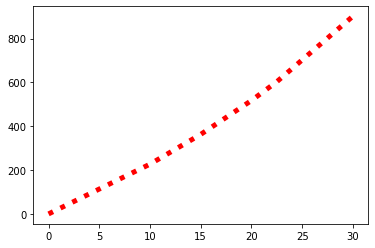##### keyword input argument linewidth¶

The linewidth is an int or a float. It is specified in points (which is 1/72 of an inch). Changing it to 72 should therefore display a line width of an inch.

In :
%matplotlib inline
plt.plot(xa,ya,color='r',linestyle='-',linewidth=72)

Out:
[<matplotlib.lines.Line2D at 0x2bee0e9d6d0>]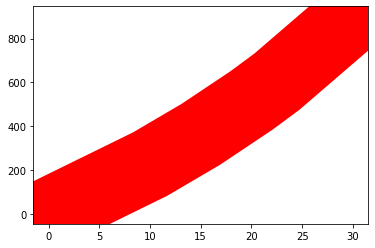It is more common to use around 3 to 5.

In :
%matplotlib inline
plt.plot(xa,ya,color='r',linestyle='-',linewidth=3)
plt.plot(xa,ya+100,color='g',linestyle='-',linewidth=4)
plt.plot(xa,ya+200,color='b',linestyle='-',linewidth=5)

Out:
[<matplotlib.lines.Line2D at 0x2bee0efe580>]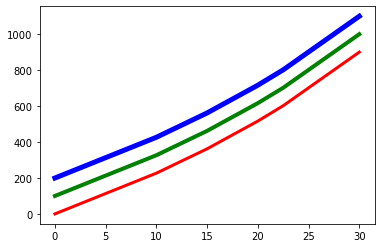##### keyword input argument color¶

We see light with our eyes and our brain translates the light intensity ratio to a color.

The eye was short, medium and long wavelength cones. The typical physiological response of the eye is shown below.We can make LEDs of these three colors.When we overlay them and vary the intensity we can use color mixing to make any color we want:

• The secondary colors cyan, magenta and yellow appear when two primary colors show full overlap
• White appears when all three primary colors show full output.
• Black is the absense of light.Color pickers in programs such as Microsoft Word use RGB values and Hex.The LEDs are each designed to have 8 bit encoding giving 256 levels (0-255):

• matplotlib accepts these directly as a [r,g,b] float. In the case of the numbers shown in word we would need to divide each through by 256.
• The [r,g,b,a] float has an additional 4th channel, the alpha channel which is a metric of transparency. (0 is completely transparent and 1 is not transparent at all).
• Hexadecimal uses the 16 characters 0,1,2,3,4,5,6,7,8,9,a,b,c,d,e,f opposed to 10 characters 0,1,2,3,4,5,6,7,8,9 in decimal. Essentially 2 hexadecimal characters can be used to designate 256 levels (16*16=256).

There is also a 1 letter and full name str for each primary color, secondary color as well as white and black.

1 letter str letter str hexadecimal str [r,g,b,a] float
'r' 'red' '#FF0000' [255/255,0/255,0/255,1]
'g' 'green' '#00FF00' [0/255,255/255,0/255,1]
'b' 'blue' '#0000FF' [0/255,0/255,255/255,1]
'c' 'cyan' '#0000FF' [0/255,255/255,255/255,1]
'y' 'yellow' '#FFFF00' [255/255,255/255,0/255,1]
'k' 'black' '#000000' [0/255,0/255,0/255,1]
'w' 'white' '#FFFFFF' [255/255,255/255,255/255,1]
In :
%matplotlib inline
plt.plot(x,y,color='#7030A0',linestyle=':',linewidth=5)

Out:
[<matplotlib.lines.Line2D at 0x2bee0f50fa0>]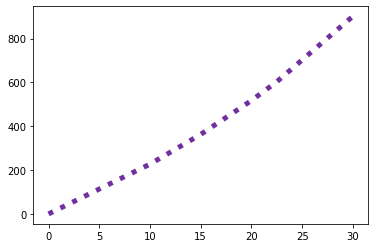In :
%matplotlib inline
plt.plot(x,y,color=[112/255,48/255,160/255],linestyle=':',linewidth=5)

Out:
[<matplotlib.lines.Line2D at 0x2bee092e220>]In :
%matplotlib inline
plt.plot(x,y,color=[112/255,48/255,160/255,0.4],linestyle=':',linewidth=5)

Out:
[<matplotlib.lines.Line2D at 0x2bee0e1cfa0>]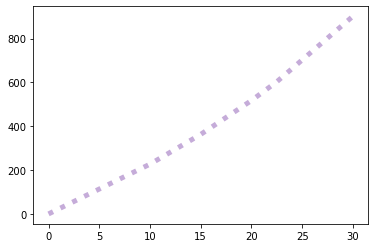##### keyword input argument linestyle¶

The keyword input argument linestyle has a 1-2 letter str that is symbolic of the line style and a str that is the name of the line style.

short str str
'-' 'solid'
'_' 'dashed'
'-.' 'dashdot'
':' 'dotted'
In :
%matplotlib inline
plt.plot(x,y,color='r',linestyle='solid',linewidth=3)
plt.plot(xa,ya+100,color='g',linestyle='dashed',linewidth=3)
plt.plot(xa,ya+200,color='b',linestyle='dashdot',linewidth=3)
plt.plot(xa,ya+300,color='k',linestyle='dotted',linewidth=3)

Out:
[<matplotlib.lines.Line2D at 0x2bee1032af0>]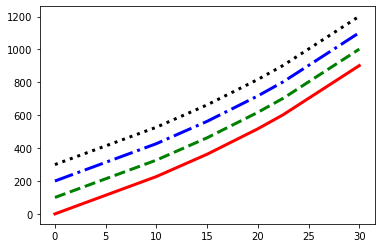##### marker keyword input arguments¶

By default no marker is displayed on the line. There are 7 marker related keyword input arguments.

In :
%matplotlib inline
plt.plot(x,y,color=[112/255,48/255,160/255],linestyle=':',linewidth=5,
marker='*',markersize=50,
markeredgewidth=5,markeredgecolor='#FF0000',
fillstyle='left',markerfacecolor='#00B050',markerfacecoloralt='#FFFF00')

Out:
[<matplotlib.lines.Line2D at 0x2bee10906d0>]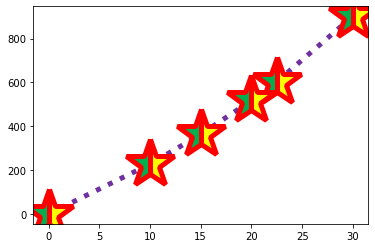The keyword marker can be toggled to.

short str description
'.' point
',' pixel
'o' circle
'v' triangle_down
'^' triangle_up
'<' triangle_left
'>' triangle_right
'1' tri_down
'2' tri_up
'3' tri_left
'4' tri_right
's' square
'p' pentagon
'*' star
'h' hexagon1
'H' hexagon2
'+' plus
'x' x
'D' diamond
'd' thin_diamond
'|' vline
'_' hline

markersize and markeredgewidth are in pt (similar to linewidth).

The fillstyle can be the following. top, bottom, left and right have an alternative marker edge color.

fillstyle
'full'
'top'
'bottom'
'left'
'right'
In :
%matplotlib inline
plt.plot(xa,ya,color=[112/255,48/255,160/255],linestyle=':',linewidth=5,
marker='*',markersize=50,
markeredgewidth=5,markeredgecolor='#FF0000',
fillstyle='left',markerfacecolor='#00B050',markerfacecoloralt='#FFFF00')
plt.plot(xa,ya+1000,color=[112/255,48/255,160/255],linestyle=':',linewidth=5,
marker='o',markersize=50,
markeredgewidth=5,markeredgecolor='#FF0000',
fillstyle='right',markerfacecolor='#00B050',markerfacecoloralt='#FFFF00')
plt.plot(xa,ya+2000,color=[112/255,48/255,160/255],linestyle=':',linewidth=5,
marker='D',markersize=50,
markeredgewidth=5,markeredgecolor='#FF0000',
fillstyle='top',markerfacecolor='#00B050',markerfacecoloralt='#FFFF00')

Out:
[<matplotlib.lines.Line2D at 0x2bee10ff0d0>]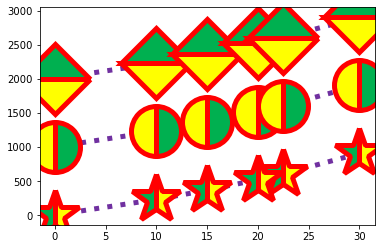##### label and legend¶

The keyword input argument label is designed to label each line plot. The labels will be automatically used when the function legend is called.

In :
%matplotlib inline
plt.plot(xa,ya,color='r',linestyle='solid',linewidth=3,label='rocket1')
plt.plot(xa,ya+100,color='g',linestyle='solid',linewidth=3,label='rocket2')
plt.plot(xa,ya+200,color='b',linestyle='solid',linewidth=3,label='rocket3')
plt.legend()

Out:
<matplotlib.legend.Legend at 0x2bee1155c70>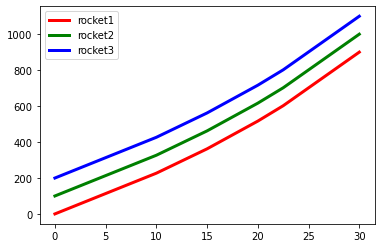We can have a look at the input arguments of the function legend by typing it in and then pressing shift ⇧ and tab ↹.We see we can use the keyword input argument labels and then provide a list of str equal to the number of plots on the figure.

In :
rlabels=['Rocket 1','Rocket 2','Rocket 3']
%matplotlib inline
plt.plot(xa,ya,color='r',linestyle='solid',linewidth=3,label='rocket1')
plt.plot(xa,ya+100,color='g',linestyle='solid',linewidth=3,label='rocket2')
plt.plot(xa,ya+200,color='b',linestyle='solid',linewidth=3,label='rocket3')
plt.legend(labels=rlabels)

Out:
<matplotlib.legend.Legend at 0x2bee1066b20>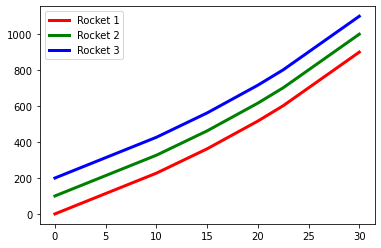We can also use the keyword loc to override the position of the legend. The default value is 'best'.

str
'best'
'upper right'
'upper left'
'lower left'
'lower right'
'right'
'center left'
'center right'
'lower center'
'upper center'
'center'

For example we can move it to the lower right.

In :
rlabels=['Rocket 1','Rocket 2','Rocket 3']
%matplotlib inline
plt.plot(xa,ya,color='r',linestyle='solid',linewidth=3,label='rocket1')
plt.plot(xa,ya+100,color='g',linestyle='solid',linewidth=3,label='rocket2')
plt.plot(xa,ya+200,color='b',linestyle='solid',linewidth=3,label='rocket3')
plt.legend(labels=rlabels,loc='lower right')

Out:
<matplotlib.legend.Legend at 0x2bee10c2f10>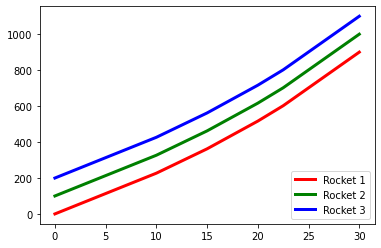#### grid¶

Multiple different plot types can be added to the figure axes. We have so far only used the single plot type plot which creates a line plot.

A grid plot type is commonly used with it to display gridlines (this plot type has no legend). To get details about the input arguments type in the function and press shift ⇧ and tab ↹.Once again the docstring is quite long so we can get the full output in a cell using.

In :
? plt.grid

Signature:  plt.grid(b=None, which='major', axis='both', **kwargs)
Docstring:
Configure the grid lines.

Parameters
----------
b : bool or None, optional
Whether to show the grid lines. If any *kwargs* are supplied,
it is assumed you want the grid on and *b* will be set to True.

If *b* is *None* and there are no *kwargs*, this toggles the
visibility of the lines.

which : {'major', 'minor', 'both'}, optional
The grid lines to apply the changes on.

axis : {'both', 'x', 'y'}, optional
The axis to apply the changes on.

**kwargs : .Line2D properties
Define the line properties of the grid, e.g.::

grid(color='r', linestyle='-', linewidth=2)

Valid keyword arguments are:

Properties:
agg_filter: a filter function, which takes a (m, n, 3) float array and a dpi value, and returns a (m, n, 3) array
alpha: float or None
animated: bool
antialiased or aa: bool
clip_box: .Bbox
clip_on: bool
clip_path: Patch or (Path, Transform) or None
color or c: color
contains: unknown
dash_capstyle: {'butt', 'round', 'projecting'}
dash_joinstyle: {'miter', 'round', 'bevel'}
dashes: sequence of floats (on/off ink in points) or (None, None)
data: (2, N) array or two 1D arrays
drawstyle or ds: {'default', 'steps', 'steps-pre', 'steps-mid', 'steps-post'}, default: 'default'
figure: .Figure
fillstyle: {'full', 'left', 'right', 'bottom', 'top', 'none'}
gid: str
in_layout: bool
label: object
linestyle or ls: {'-', '--', '-.', ':', '', (offset, on-off-seq), ...}
linewidth or lw: float
marker: marker style string, ~.path.Path or ~.markers.MarkerStyle
markeredgecolor or mec: color
markeredgewidth or mew: float
markerfacecolor or mfc: color
markerfacecoloralt or mfcalt: color
markersize or ms: float
markevery: None or int or (int, int) or slice or List[int] or float or (float, float) or List[bool]
path_effects: .AbstractPathEffect
picker: unknown
rasterized: bool or None
sketch_params: (scale: float, length: float, randomness: float)
snap: bool or None
solid_capstyle: {'butt', 'round', 'projecting'}
solid_joinstyle: {'miter', 'round', 'bevel'}
transform: matplotlib.transforms.Transform
url: str
visible: bool
xdata: 1D array
ydata: 1D array
zorder: float

Notes
-----
The axis is drawn as a unit, so the effective zorder for drawing the
grid is determined by the zorder of each axis, not by the zorder of the
.Line2D objects comprising the grid.  Therefore, to set grid zorder,
use .set_axisbelow or, for more control, call the
~.Artist.set_zorder method of each axis.
File:      c:\users\phili\anaconda3\lib\site-packages\matplotlib\pyplot.py
Type:      function


We see the main keyword input arguments are b which is a bool (True or False), which (has the str options 'major' and 'minor' and axis (has the str options 'x' and 'y').

b has the default value of True meaning a default grid will be shown if it is enabled. We see the same behaviour if we call the function grid without specifying any keyword input arguments or if we explicitly set b to True.

In :
%matplotlib inline
plt.plot(xa,ya,color='r',linestyle='solid',linewidth=3,label='rocket1')
plt.legend()
plt.grid()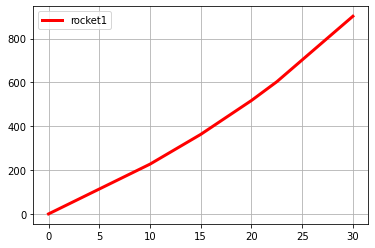In :
%matplotlib inline
plt.plot(xa,ya,color='r',linestyle='solid',linewidth=3,label='rocket1')
plt.legend()
plt.grid(b=True)Setting b to False shows no grid as expected.

In :
%matplotlib inline
plt.plot(xa,ya,color='r',linestyle='solid',linewidth=3,label='rocket1')
plt.legend()
plt.grid(b=False)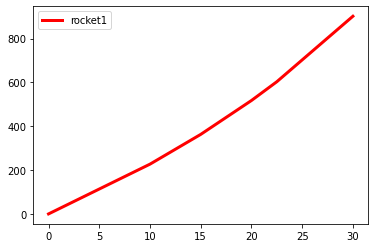The keyword argument axis has the default value of both (meaning both x and y gridlines are shown). We see the same behaviour if we call the function axis without specifying any keyword input arguments or if we explicitly set axis to 'both'.

In :
%matplotlib inline
plt.plot(xa,ya,color='r',linestyle='solid',linewidth=3,label='rocket1')
plt.legend()
plt.grid()In :
%matplotlib inline
plt.plot(xa,ya,color='r',linestyle='solid',linewidth=3,label='rocket1')
plt.legend()
plt.grid(axis='both')Alternatively we can set it to only 'x' or 'y' respectively.

In :
%matplotlib inline
plt.plot(xa,ya,color='r',linestyle='solid',linewidth=3,label='rocket1')
plt.legend()
plt.grid(axis='x')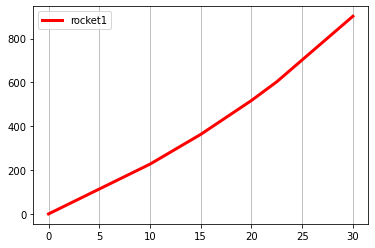In :
%matplotlib inline
plt.plot(xa,ya,color='r',linestyle='solid',linewidth=3,label='rocket1')
plt.legend()
plt.grid(axis='y')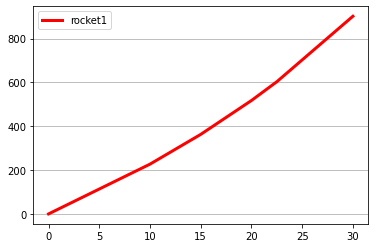The keyword argument which is set to major by default which shows the grid only at the numbers displayed in the axis.

In order to also display minor ticks we need to use the function minorticks_on (there is also the function minorticks_off which will turn them off). We can press shift ⇧ and tab ↹ to get details about the input arguments we see there are none.With the minor ticks on we see that there are 4 minor ticks for each major tick.

In :
%matplotlib inline
plt.plot(xa,ya,color='r',linestyle='solid',linewidth=3,label='rocket1')
plt.legend()
plt.minorticks_on()
plt.grid()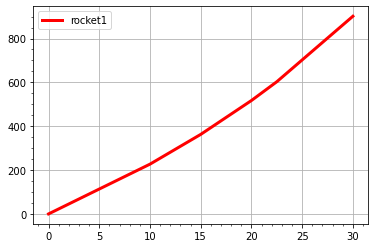We can also gridlines for these by selecting which='minor'. Or if we want both the minor and major gridlines to have the same style we can set which='both'.

In :
%matplotlib inline
plt.plot(xa,ya,color='r',linestyle='solid',linewidth=3,label='rocket1')
plt.legend()
plt.minorticks_on()
plt.grid(which='minor')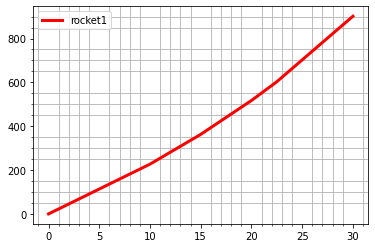In :
%matplotlib inline
plt.plot(xa,ya,color='r',linestyle='solid',linewidth=3,label='rocket1')
plt.legend()
plt.minorticks_on()
plt.grid(which='both')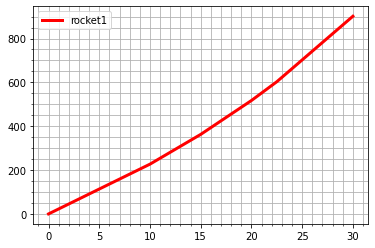There are a number of additional keyword input arguments however the ones color, alpha, linestyle and linewidth are most commonly used. These act similar to the equivalent keyword arguments seen in the plot function. Let's use them to create a custom grid with major and minor grid lines.

In :
%matplotlib inline
plt.plot(xa,ya,color='r',linestyle='solid',linewidth=3,label='rocket1')
plt.legend()
plt.grid(which='major',color='#00B050',linestyle='-',linewidth=0.8,alpha=0.8)
plt.minorticks_on()
plt.grid(which='minor',color='#999999',linestyle=':',linewidth=0.5,alpha=0.5)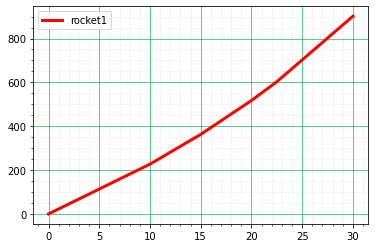In :
%matplotlib inline
plt.plot(xa,ya,color='r',linestyle='solid',linewidth=3,label='rocket1')
plt.legend()
plt.grid(which='major',color='#999999',linestyle='-',linewidth=0.8,alpha=0.8)
plt.minorticks_on()
plt.grid(which='minor',color='#999999',linestyle=':',linewidth=0.5,alpha=0.5)
plt.xlabel('time (s)')
plt.ylabel('velocity (m/s)')
plt.title('Velocity of a Rocket')

Out:
Text(0.5, 1.0, 'Velocity of a Rocket')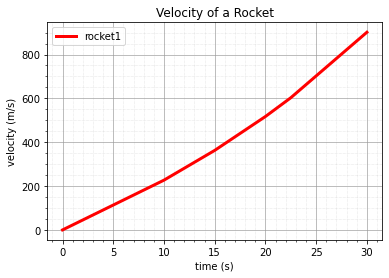In :
%matplotlib inline
plt.plot(xa,ya,color='r',linestyle='solid',linewidth=3,label='rocket1')
plt.legend()
plt.grid(which='major',color='#999999',linestyle='-',linewidth=0.8,alpha=0.8)
plt.minorticks_on()
plt.grid(which='minor',color='#999999',linestyle=':',linewidth=0.5,alpha=0.5)
plt.xlabel('time (s)')
plt.ylabel('velocity (m/s)')
plt.title('Velocity of a Rocket')
plt.show()#### tick params¶

The tick parameters can be changed using the function tick_params. It shares the keyword input arguments axis and which with grid.The docstring is relatively stron we can view the full output in a cell.

In :
? plt.tick_params

Signature:  plt.tick_params(axis='both', **kwargs)
Docstring:
Change the appearance of ticks, tick labels, and gridlines.

Tick properties that are not explicitly set using the keyword
arguments remain unchanged unless *reset* is True.

Parameters
----------
axis : {'x', 'y', 'both'}, default: 'both'
The axis to which the parameters are applied.
which : {'major', 'minor', 'both'}, default: 'major'
The group of ticks to which the parameters are applied.
reset : bool, default: False
Whether to reset the ticks to defaults before updating them.

Other Parameters
----------------
direction : {'in', 'out', 'inout'}
Puts ticks inside the axes, outside the axes, or both.
length : float
Tick length in points.
width : float
Tick width in points.
color : color
Tick color.
Distance in points between tick and label.
labelsize : float or str
Tick label font size in points or as a string (e.g., 'large').
labelcolor : color
Tick label color.
colors : color
Tick color and label color.
zorder : float
Tick and label zorder.
bottom, top, left, right : bool
Whether to draw the respective ticks.
labelbottom, labeltop, labelleft, labelright : bool
Whether to draw the respective tick labels.
labelrotation : float
Tick label rotation
grid_color : color
Gridline color.
grid_alpha : float
Transparency of gridlines: 0 (transparent) to 1 (opaque).
grid_linewidth : float
Width of gridlines in points.
grid_linestyle : str
Any valid .Line2D line style spec.

Examples
--------
::

ax.tick_params(direction='out', length=6, width=2, colors='r',
grid_color='r', grid_alpha=0.5)

This will make all major ticks be red, pointing out of the box,
and with dimensions 6 points by 2 points.  Tick labels will
also be red.  Gridlines will be red and translucent.
File:      c:\users\phili\anaconda3\lib\site-packages\matplotlib\pyplot.py
Type:      function


The keyword input arguments axis and which share commonalities with their counterparts in the function grid. We can also specify the direction (using the str 'in', 'out' or 'both', length and width (as ints or flaots), color (of the ticks), labelcolor and labelsize (of the tick labels - not of the xlable or ylabel).

We can specify the locations to display the ticks (left, right, top, bottom) and the tick labels (labelleft, labelright, labeltop, labelbottom). These are Boolean values

In :
%matplotlib inline
plt.plot(xa,ya,color='r',linestyle='solid',linewidth=3,label='rocket1')
plt.legend()
plt.grid(which='major',color='#999999',linestyle='-',linewidth=0.8,alpha=0.8)
plt.minorticks_on()
plt.grid(which='minor',color='#999999',linestyle=':',linewidth=0.5,alpha=0.5)
plt.xlabel('time (s)')
plt.ylabel('velocity (m/s)')
plt.title('Velocity of a Rocket')
plt.tick_params(axis='x',which='major',
direction='in',length=10,width=3,
color='r',labelcolor='b',
labelsize=12,top=True,bottom=True,
labeltop=True,labelbottom=True)
plt.tick_params(axis='y',which='major',
direction='out',length=10,width=3,
color='c',labelcolor='m',
labelsize=12,left=True,right=True,
labelleft=True,labelright=True)
plt.show()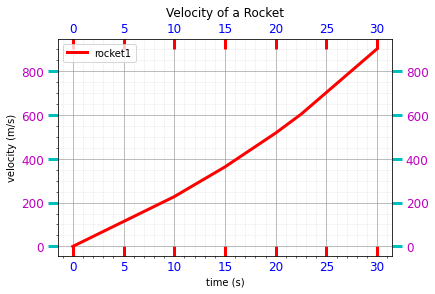#### axhline, axvline, hlines and vlines¶

Sometimes we may want to place an emphasize on a certain point by drawing either a horizontal line to it or a vertical line to it. This can be done using the functions axhline and axvline. We can get details about the keyword input arguments for each of the function names by pressing shift ⇧ and tab ↹.

We seen the horizontal line is added fully along the x-axis by default. We can overrride this by selecting the value of y and the xmin and xmax when we want to draw the horizontal line partially along the axis.We seen the vertical line is added fully along the y-axis by default. We can overrride this by selecting the value of x and the ymin and ymax when we want to draw the vertical line partially along the axis.The other keyword input arguments color. alpha, linestyle and linewidth act in the same manner as their counterparts within the plot and grid functions.

For example if we want to emphasize the point x=20,y=517.35. We can do this using the axline and axvline.

In :
%matplotlib inline
plt.plot(xa,ya,color='r',linestyle='solid',linewidth=3,label='rocket1')
plt.legend()
plt.grid(which='major',color='#999999',linestyle='-',linewidth=0.8,alpha=0.8)
plt.minorticks_on()
plt.grid(which='minor',color='#999999',linestyle=':',linewidth=0.5,alpha=0.5)
plt.xlabel('time (s)')
plt.ylabel('velocity (m/s)')
plt.title('Velocity of a Rocket')
plt.axhline(y=517.35,color='#000000',linestyle='-',linewidth=2,alpha=1)
plt.axvline(x=20,color='#000000',linestyle='-',linewidth=2,alpha=1)
plt.show()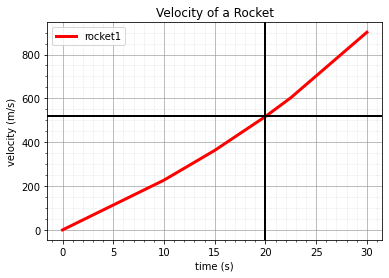If we only want it to the draw the point partially along the axes we can specify xmin, xmax, ymin and ymax which are all normalized floats.

In :
%matplotlib inline
plt.plot(xa,ya,color='r',linestyle='solid',linewidth=3,label='rocket1')
plt.legend()
plt.grid(which='major',color='#999999',linestyle='-',linewidth=0.8,alpha=0.8)
plt.minorticks_on()
plt.grid(which='minor',color='#999999',linestyle=':',linewidth=0.5,alpha=0.5)
plt.xlabel('time (s)')
plt.ylabel('velocity (m/s)')
plt.title('Velocity of a Rocket')
plt.axhline(y=517.35,xmin=0.25,xmax=0.75,color='#000000',linestyle='-',linewidth=2,alpha=1)
plt.axvline(x=20,ymin=0.25,ymax=0.75,color='#000000',linestyle='-',linewidth=2,alpha=1)

Out:
<matplotlib.lines.Line2D at 0x2bee5333c70>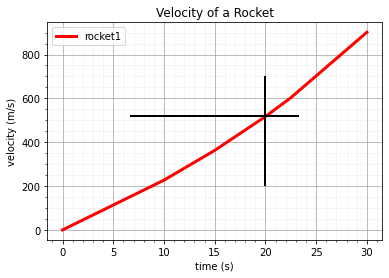If we want to instead specify the lines to a datapoint we should instead use the methods hlines and vlines. We can get details about the keyword input arguments for each of the function names by pressing shift ⇧ and tab ↹. We see that they are similar to axhline and axvline.In :
%matplotlib inline
plt.plot(xa,ya,color='r',linestyle='solid',linewidth=3,label='rocket1')
plt.legend()
plt.grid(which='major',color='#999999',linestyle='-',linewidth=0.8,alpha=0.8)
plt.minorticks_on()
plt.grid(which='minor',color='#999999',linestyle=':',linewidth=0.5,alpha=0.5)
plt.xlabel('time (s)')
plt.ylabel('velocity (m/s)')
plt.title('Velocity of a Rocket')
plt.hlines(y=517.35,xmin=0,xmax=20,color='#000000',linestyle='-',linewidth=2,alpha=1)
plt.vlines(x=20,ymin=0,ymax=517.35,color='#000000',linestyle='-',linewidth=2,alpha=1)

Out:
<matplotlib.collections.LineCollection at 0x2bee53e2f40>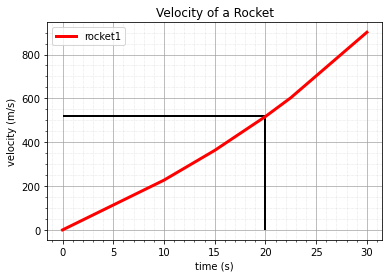Note the funtion is plural and the keyword input arguments can be singular or plural. We can use hlines for example to draw three vertical lines. This may be used to emphasize uncertainties in a datapoint for example.

In :
%matplotlib inline
plt.plot(xa,ya,color='r',linestyle='solid',linewidth=3,label='rocket1')
plt.legend()
plt.grid(which='major',color='#999999',linestyle='-',linewidth=0.8,alpha=0.8)
plt.minorticks_on()
plt.grid(which='minor',color='#999999',linestyle=':',linewidth=0.5,alpha=0.5)
plt.xlabel('time (s)')
plt.ylabel('velocity (m/s)')
plt.title('Velocity of a Rocket')
plt.hlines(y=[490,517.35,550],xmin=0,xmax=20,colors=['#0000FF','#000000','#0000FF'],linestyles=[':','-',':'],linewidths=[2,4,2],alpha=1)

Out:
<matplotlib.collections.LineCollection at 0x2bee5145670>